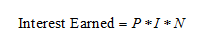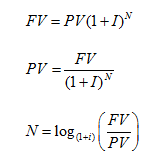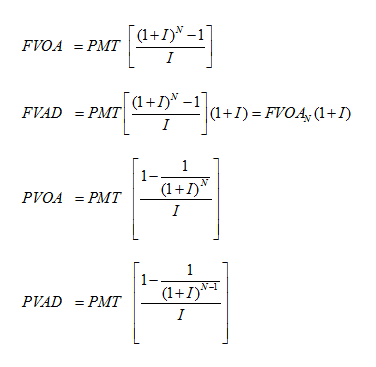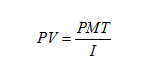# Time Value of Money

Time Value of Money is the concept that money today may be worth something different at another point in time. The two things that influence the value of money over time are interest and inflation, both of which can be calculated with the equations presented here. When making monetary decisions it is import to be able to compare today’s dollar against tomorrow’s dollar.

Inflation is the rise in price of goods and services over a period of time.

Interest is the cost for the use of money. It is the amount paid above and beyond what is borrowed or lent (Principal). An established business practice is to express interest as a percentage rate. It is also usually required to disclose an interest rate on an annual basis.

### Variables involved in calculating interest

1. PRINCIPAL: The amount borrowed or lent
2. INTEREST RATE: The ratio to principal that must be paid for its use.
3. TIME: The duration that the principle is outstanding.

### Simple Interest

Simple interest is earned when past interest is not added to principle in future periods for computing interest.Where P = Principal I = rate of interest per period N = number of periods

### Compound Interest

Compound interest is earned on the initial principal and the accumulated interest of the initial principal, assuming the interest is left on deposit. Compounding interest will be calculated on the accumulated balance. Principal plus interest earned, at the end of a period.Where PV = Present Value FV = Future Value I = rate of interest per period N = number of periods

### Annuity

An annuity is a series of equal payments at fixed intervals for a specified number of periods. An ordinary (deferred) annuity is an annuity whose payments occur at the end of each period. An annuity due is an annuity whose payments occur at the beginning of each period.Where FVOA = Future Value Ordinary Annuity FVAD = Future Value Annuity Due PVOA = Present Value Ordinary Annuity PVAD = Present Value Annuity Due PMT = Payment I = rate of interest per period N = number of periods

To find the value of a long term bond is a three-step process. 1) find the present value of the bond at maturity 2) find the present value of periods interest payments 3) add them together

### Perpetuities

Similar to an annuity a perpetuity is a stream of equal payments at fixed intervals expected to continue forever.Where PV = Present Value PMT = Payment I = rate of interest per period

### Amortized Loans

An amortized loan has scheduled periodic payments of both principal and interest, that is repaid in equal amounts on a predetermined schedule; usually monthly, quarterly or annually . This is opposed to loans with interest-only payment features.